×
Get Full Access to UGA - STAT 2000 - Study Guide - Midterm
Get Full Access to UGA - STAT 2000 - Study Guide - Midterm

×

UGA / Engineering / STAT 2000 / What is statistics?

# What is statistics? Description

Test 1 Study Guide

## What is statistics?Chapter 1: Learning from Data

1.1 Defining Statistics

• Statistics: a way of reasoning, along with a collection of tools methods, designed to help us  understand the world

• Statistical Method

o Design: how the data are obtained (using a survey, setting up an experiment); valid  inferences cannot be made without a good design

o Description: summarizing the data obtained (providing mean or percentage,  creating bar graph or pie chart); can help identify patterns in the data Don't forget about the age old question of if an atom has 15 protons how many electrons does it have

o Inference: making decisions or predictions based on the data

• Our ability to answer questions and draw conclusions from data depends largely on our  ability to understand variation; key to learning from data is understanding the variation  that is all around us

## What are the statistical methods?• Statistics: a field of study; science of learning from data

o What are data?

▪ Information – could be numbers (weight, income) or words (gender, race) o How do we learn from it?

▪ Involves analyzing data and making inferences

1.2 Populations and Samples

• Population: set of all individuals we are interested in studying

o Studying an entire population can be difficult (size, cost, time-consuming) so  samples are often studied instead

• Sample: subset of a population for whom we have data

o Subjects: things or individuals who make up the sample

## What are the basic ways to gather data?If you want to learn more check out kin 351 study guide

o Ideally, characteristics of the subjects in the sample will tell us something about the  overall population

Population

Sample

Subjects

The entire voting public

200 randomly selected

voters

Each voter in the sample

Every MLB player

60 randomly selected

players

Each player in the sample

All 159 counties in Georgia

40 randomly selected

counties

Each county in the sample

• Parameter: numerical value summarizing the population data

o Value is usually unknown or unknowable

o Population mean or population proportion

• Statistics: numerical value summarizing the sample data

o If we have data, we know the statistics

o Sample statistics serve as estimates for population parameters

• Using symbols

Value

Symbol

How to say it

Population mean (parameter)

mu

Sample mean (statistic)

x-bar

Population proportion

(parameter)

p

P

Sample proportion (statistic)

p-hat

Sample size

n

n

Don't forget about the age old question of ○ Identification (individualization) → how do we know an object retains its identity when it is unseen?

• Proportion: another way to express a percentage; must be between 0 and 1 o 50% is 0.50, 95% is 0.95, 5% is 0.05

o Typically used instead of percentages Don't forget about the age old question of comm 2500 class notes

Chapter 4: Gathering Data

4.1 Experimental and Observational Studies

• Basic ways to gather data:

1. Conduct an experiment

2. Observational study

3. (Census)

• Experiments: attempt to manipulate or influence the subjects in order to obtain the data.  o Subjects are usually randomly assigned to groups – a control group and a treatment  group Don't forget about the age old question of How does tolerance for variation in body temperature vary among plants, ectothermic animals, and endothermic animals?

o If properly designed, can be used to show causation

• Observational study: measures the characteristics of the subjects without attempting to  manipulate or influence the subjects

o Observes rather than experiments

o Cannot conclude causation, but can conclude that two variables are related;  correlation does not mean causation

• We can study the effect of an explanatory variable (x-axis) on a response variable (y-axis) with an experiment than with an observational study

o With an experiment, causation might be a valid conclusion

4.2 Good and Poor Ways to Sample

• Goal in observational studies is not only to describe the sample that we see, but hopefully to  generalize characteristics of the sample to a much large population of individuals • When population is too large for us to gather data on each and every individual, we rely on  sample to tell us about the population

o Only works when sample is representative of population

• Other Types of Sampling Methods

Type of Sampling

Description

Simple random

sampling

Each subject in population has same chance of being included  in sample. (Each possible sample of a certain size has same  chance of being selected.)

Stratified sampling

Population is divided into non-overlapping, homogeneous  groups (called strata) and a simple random sample is then  obtained from each group.

Cluster sampling

Population is divided into non-overlapping, heterogeneous groups and all individuals within a randomly selected group or  groups are sampled; each cluster represents population

Convenience sampling

Sampling where individuals are easily obtained. For example,  internet surveys. Not the best option

Systematic sampling

Using a rule to select a sample. For example, selecting every  10th person from the population.

o Best sampling method is simple random sampling

▪ Most likely to get a good reflection of the characteristics of the members of  the population through random sampling

o Difference between stratified and cluster sampling

▪ Stratified: samples some individuals from all groups

▪ Cluster: samples all individuals from some groups

o Key to all good sampling methods is involving chance and eliminating personal  choices

• Many observational studies involve surveys

• Important to avoid bias in surveys

o Bias: the sample data are not representative of the population

o Ex: the results of an opinion survey asking about raising the sales tax to support  public schools may be biased if you sample only educators

• Sources of potential bias in surveys

o Sampling bias: using nonrandom samples or from undercoverage, which means  parts of the population are not represented

▪ Example in paragraph above illustrates undercoverage because we can’t  learn about the entire population if we only sample educators

o Nonresponse bias: some sampled subjects cannot be reached or refuse to  participate; the subjects that are willing to participate may have strong emotional  convictions about the issue being surveyed and would not represent the population  well

▪ Ex: if we send out 500 surveys and only get 20 back, this could result in  nonresponse bias

o Response bias: subject gives an incorrect response (lying) or when the questions  are asked in confusing or misleading ways

▪ Ex: wording a survey question like this “Don’t you agree that candidate A is  better than candidate B who has a history of dishonesty?” This question is

leading respondents to agree with the statement.

o Poor ways to sample: convenience and volunteer

▪ Convenience samples: convenient and therefore easy but typically, they  don’t represent the population

▪ When respondents volunteer to participate, individuals with the strongest  feelings on either side of an issue are more likely to respond; those who

don’t care may not bother, creating a voluntary response bias

• NOTE: A large sample does NOT remove nor reduce bias. If the process is inherently flawed,  doing that same process more just results in more biased data.

• Generalizing results from the sample to the population (random sampling) o Simple random sampling (and probability sampling methods in general) allow us to  believe that our sample is representative of the larger population and that the  observed sample statistic is “in the ball park” of the population parameter.  ▪ We will be willing to generalize the characteristics of our sample to the  entire population

▪ How large is the ballpark? How far do we think anyone observed statistic  could be from the parameter?

4.3 Good and Poor Ways to Experiment

• Experimental unit (or subject): is the person or object upon which a treatment is applied • Treatment: a condition applied to the subject (a new drug, perhaps)

• Explanatory variable: explains or influences changes in a response variable • Response variable: variable of interest; what we measure after treatments are applied • Controlled study: treatments are randomly applied to experimental units

o Goal of an experiment is to determine the effect the treatment has on the response  variable

• Random assignment: necessary to even out the effects of other variables, known and  unknown

o To coin a phrase, “control for the variables you can, randomize for all others.” • In medical experiments to test the effects of new drugs, researchers often administer either  (1) a dose of the new drug or (2) a placebo to each patient

o Placebo: a “dummy” treatment; in this case, it’s a fake pill

o Patient does not know if they are getting the real medication or not

o Outcomes in treatment group will be compared with outcomes in the control group  (those who received the placebo)

• Double blind: researchers don’t know which patients are getting the real medication and  neither will the patients

• Single blind: researcher does know which patients got the real medication • Treatments are randomly assigned to subjects (or subjects to treatments) at random o Purpose of random assignment is to reduce bias and even out the effect of other  variables

o Confounding: two explanatory variables are both associated with a response  variable

4.4 Other Ways to Conduct Experimental Studies

• Types of Experimental Designs

o Completely randomized design: experimental units are randomly assigned to the  treatment

o Matched-pairs design: experimental units are related (twins, husband and wife) or  matched before the experiment takes place; measure of the response variable is  taken before a treatment is applied and then the same measure is taken from the

same subject after the treatment; having before and after measurements from one  person is a common matched-pairs design

o Sometimes, it’s necessary to group similar subjects based on one particular attribute ▪ Blocking: using a factor to create homogeneous groups (blocks)

▪ All experimental conditions are then tried in each block

• A side note on matched-pairs designs

o Ex: randomly select 20 students and have them take both Test A and Test B ▪ Matching Test A scores against Test B scores for the same students

▪ Experimental units in each group are related because they are the same  students

o Not an example: randomly select 40 students and randomly divide them into two  groups of 20 where each group will take a different test

▪ Can’t compare test scores for each individual, but we could still compare  average Test A score to average Test B score

• Causation: can we say what caused the effect? (Random Assignment)

o Can think of science as a search for cause-and-effect relationships and for theories  that unite them

o Initially, scientists found that smokers had higher rates of lung cancer than did non smokers; smoking and cancer were associated

▪ Did that prove that smoking caused cancer? No

o Ex: some scientists thought there might be a gene that made people both likely to  smoke and likely to get lung cancer

▪ Presence of gene could be a confounding variable (association)

o To conclude that smoking causes lung cancer, you must be able to rule out the effect  of possible confounders

▪ Randomized experiments accomplish this

▪ If a randomized experiment finds an effects, we can conclude the difference  in treatments caused the effect

o Is association evidence of possible causation? Yes

▪ If smoking causes cancer, there has to be an association

▪ Cancer rates will be higher for smokers

▪ If the two are associated, one might cause the other

▪ Association is necessary, but association alone is not enough to prove cause and-effect

• Summarize Random Sampling vs. Random Assignment

o Well-designed experiment uses random assignment to determine which  observational (experimental) units go into which explanatory variable groups ▪ Goal: to produce groups that are similar as possible in all respects except for  the explanatory variable

▪ If the groups can be shown to differ significantly on the response variable,  the difference can be attributed to a cause-and-effect relationship between  the explanatory and response variables

o Random assignment: a very different use of randomness from random sampling,  with different implications for scope of conclusions

▪ Aims to select a representative sample from a population, so that results  about the sample can be generalized to the larger population

▪ Aims to produce similar treatment groups, so that a significant difference in  the response variable between groups can be attributed (causally) to the

explanatory variable

Chapter 2: Exploring and Summarizing Data

• Variable: any characteristic we are studying – height, GPA, religious affiliation, income,  major, and number of pets

o Expect to see a certain amount of variability

o Statistical methods provide ways to measure and understand variability 2.1 Different Types of Data

• Categorical variable: if each observation belongs to one of a set of categories • Quantitative variable: if observations are numerical values

o Discrete: have a countable number of values

o Continuous: usually variables that can take on all values on an interval

Variable Type

Examples

Categorical

Gender (male, female), type of residence (house, apartment, dorm,  other), political affiliation (Republican, Democrat, other)

Quantitative

Age, daily high temperature, SAT score, calories consumed per day

• If you’re not sure if a variable is categorical or quantitative, think about find the average o If it makes sense to find average, variable is quantitative

o If it doesn’t make sense to find average, variable is categorical

• Frequency table: a listing of possible values for a variable together with the number of  observations for each value

o Frequency means count

• Bar graphs: categories are on horizontal axis while frequencies (or proportions) are on the  vertical axis

o Heights of the rectangles for each category are equal to the category’s frequency or  proportion

o Pareto chart: bar graph with categories ordered by their frequency, from tallest bar  to shortest bar (normal bar graph)

o Comparing bar chart in descending order with vertical axis being the proportion in  each category is very similar to having frequency as the vertical axis

• Pie chart: a circle divided into sectors; each sector represents a category of data • Conditional distribution: distribution for just those cases that satisfy a specified condition • RELATIVE RISK WON’T BE ON TEST

• Dot plot: a plot with dots

o Example: 3, 7, 6, 8, 5, 5, 7, 5, 9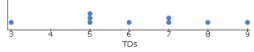o

• Histogram: a graph that uses bars to portray the frequencies of the possible outcomes of a  quantitative variable

o Horizontal (x) axis represents values the variable can take on

o Vertical (y) axis tells how many of each value falls within a certain range of value The Shape of a Distribution

• Looking at shape of a histogram (or dot plot) allows us to describe the distribution of the  data set

Shape

Description

How it looks

Symmetric/Unimodal

One side is a mirror image of the other.  The histogram looks symmetric (ex: SAT  scores, height of male UGA students)Skewed Left

Left tail is stretched out longer than the  right tail (ex: lifespan of humans)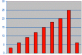Skewed Right

Right tail is stretched out longer than  the left tail (ex: income, number of pets)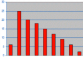Bimodal

Two distinct humps (ex: height of all  UGA students)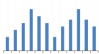• Mostly deal with first three types of graphs shown above

2.3 Measuring the Center of Quantitative Data

• Mean (average): sum of the observations divided by the number of observations • Median: the point that splits the data in two when ordered from smallest to largest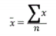• Formula for mean is: ???? summation notation

• If data is skewed, report the median

• If data is symmetrical, report the mean

• Comparing the Mean and Median

o Mean is sensitive to extreme values

▪ Sensitive: an extremely large value will pull mean to the right; extremely  small value will pull the mean to the left

o Median is generally resistant to extreme values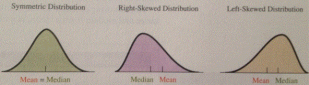2.4 Measuring the Variability of Quantitative Data

• Three measures of spread: range, IQR, & standard deviation

o Range: difference between the smallest and largest observations

2.5 Using Measures of Position to Describe Variability

• pth Percentile: a value such that p percent of the observations fall below or at that value o Ex: SAT score falls in 90th percentile ???? 90% of people scored below you • Quartiles: divide data into fourths

o First quartile: 25th percentile (Q1)

o Second quartile: 50th percentile (Q2)

▪ Median = Q2

o Third quartile: 75th percentile (Q3)

• Middle 50% of observations fall between Q1 and Q2

o 25% from Q1 to median; 25% from median to Q3

• Interquartile Range, IQR: distance from Q1 to Q3 (IQR = Q3 – Q1)

• Detecting Potential Outliers

o Outlier: unusually small or unusually large observation

▪ Can occur due to an error in data entry, but isn’t always the case

o Consider the number of home runs Brady Anderson hit per season from 1992 to  2001: 21, 13, 12, 16, 50, 18, 18, 24, 19, 8

▪ Fifty is an unusually large observation; likely classified as an outlier

o To flag an observation as potentially being an outlier, use 1.5 x IQR Criterion ▪ If an observation is less than Q1 – 1.5(IQR) or greater than Q3 + 1.5(IQR), it’s  considered an outlier

• The Five-Number Summary and Box Plots

o Five-number summary: a data set is the minimum value, the first quartile, the  median, the third quartile, and the maximum value.

▪ Basis of a graphical display called the box plot (sometimes called a box-and whisker plot due to its appearance)

• Using the Box Plot to Determine the Shape of the Distribution

o If the box plot looks approximately symmetric, then the distribution is

approximately symmetric

o If median is closer to Q1 and/or the right whisker is much longer than the left  whisker, then the distribution is skewed right.

o If the median is closer to Q3 and/or the left whisker is much longer than the right  whisker, then the distribution is skewed left.

• Symmetric• Skewed right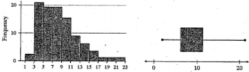• Skewed left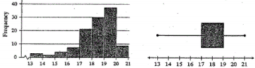• Standard deviation: describes how far observations in data set typically fall from mean o The more spread apart the numbers in the data set are, the greater the standard  deviation will be

o Variance: square the standard deviation

Summary – How to Measure and Report Center and Spread

• Five-number summary usually better than mean and standard deviation for describing a  skewed distribution or a distribution with extreme outliers.

• Use mean and standard deviation only for reasonably symmetric distributions that are free  of outliers

• Measures of center and spread for a distribution

o If shape is skewed, median and IQR should be reported

o If shape is unimodal and symmetric, mean and standard deviation and possibly  median and IQR should be reported.

o If there are unusual observations point them out and report the mean and standard  deviation with and without the values.

o Remember – The median and IQR are resistant to skewness and outliers, but the  mean and standard deviation are not.

The Empirical Rule (68-95-99.7 Rule)

• If a distribution is bell shaped, then approximately

o 68% of the observations fall within 1 standard deviation of the mean, denoted x̄± s o 95% of the observations fall within 2 standard deviations of the mean, x̄± 2s o Nearly all observations fall within 3 standard deviations of the mean, x̄± 3s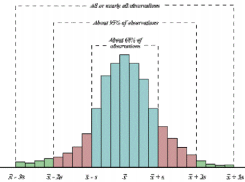o Any observations that lie outside 3 standard deviations would be outliers

The z-scale and the z-score

• Z-scale: standardized scale with a mean = 0 and a standard deviation = 1. • Z-score: tells us how many standard deviations the observation falls from the mean o Positive: indicates observation is above the mean

o Negative; indicates observation is below the mean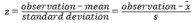???? memorize for test

Using z-scores to check for Outliers

• If data value falls more than three standard deviations from the mean, it’s regarded as an  outlier

o Z-score less than -3 or greater than +3

Chapter 3: Association – Correlation and Regression

• Response variable: variable that can be explained by, or is determined by, another variable o When two variables are quantitative, response variable will be the y-variable  (vertical axis when graphing data)

• Explanatory variable: explains, or affects, the response variable

o When two variables are quantitative, explanatory variable will be the x-variable  (horizontal axis when graphing data)

• Association: relationship exists between two variables if a particular value for one variable  is more likely to occur with certain values of the other variable

• Scatterplot: explanatory variable on horizontal axis and response variable on vertical axis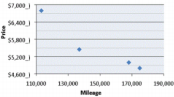o Examine to determine shape (approximately linear or not) and direction • Types of Association

o Positive association: as x increases, y tends to increase

o Negative association: as x increases, y on average decreases

o No association: as x increases, response values are scattered around with no  relationship to explanatory variable values

Linear Correlation

• When data points follow a roughly straight-line trend, variables are said to have an  approximately linear relationship

• Correlation (coefficient): denoted by symbol r

o -1 ≤ r ≤ 1

o If r is positive, the variables have a positive linear relationship (positive association) o If r is negative, the variables have a negative linear relationship

An r value close to -1 or 1 indicates a strong linear relationship. An r value close to 0 indicates a  weak linear relationship (or no linear relationship at all). Scatterplots and Correlation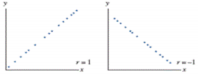• In observational studies that conclude association, there’s a possibility that some third  (lurking) variable is affecting both observed variables. Must resist temptation to conclude  that x causes y from a correlation, regardless of how strong the relationship is, regardless of  how obvious that conclusion may seem

3.3 Predicting the Outcome of a Variable

• Regression line: line that best describes linear relationship between two quantitative  variables

o y-hat = a + bx

▪ y-hat: predicted value of the response variable

▪ x: explanatory variable

▪ a: y-intercept, which is predicted value of y when x equals 0 (constant)

▪ b: slope, which is the amount that the predicted, or average, response

changes for every one unit increases in the explanatory variable (coefficient) • + = two variables have positive correlation

• – = two variables have negative correlation

▪ Can find predicted values for response variable by substituting values for x • Residual: difference between an observed value of the response variable and the value  predicted by the regression line

o Residual = actual – predicted = y – y-hat

o Positive: above regression line; Negative: below regression line

• R-squared (R2)

How can we determine if the linear model is a good fit of the data and therefore useful for  prediction? Variation in the residuals is the key to assessing how well the model fits. Total variation in response =

variation explained by model + variation NOT explained by model

R2 = variation explained by model / total variation

3.4 Cautions in Analyzing Associations

• Extrapolation: using a regression line to predict y values for x values outside observed  range of data

• Regression outliers: points that are well removed from the trend that the rest of the data  follows

o Regression line is often pulled toward this

• Correlation does not imply causation!

Page ExpiredIt looks like your free minutes have expired! Lucky for you we have all the content you need, just sign up here
References: